# ICSE Class 10 Physics Question Paper Solution 2020

Practising the ICSE Class 10 Physics Question Paper Solution 2020 helps students in their board exam preparation. They can easily evaluate their performance and analyse the areas where extra effort is required. So, to help them in their revision and prepare well for the exam, we have provided the ICSE Class 10 Physics Question Papers Solutions 2020.

The ICSE Class 10 Physics 2020 exam was conducted on 13th March 2020. The exam started at 11 am, and students were allotted 2 hours of time to complete the paper. Students can download the ICSE Class 10 Physics Question Paper Solution 2020 PDF from the link below.

Students can have a look at the ICSE Class 10 Physics Question Paper Solution 2020 below. They can also access the year wise ICSE Class 10 Physics Solved Previous Year Question Papers for more practice.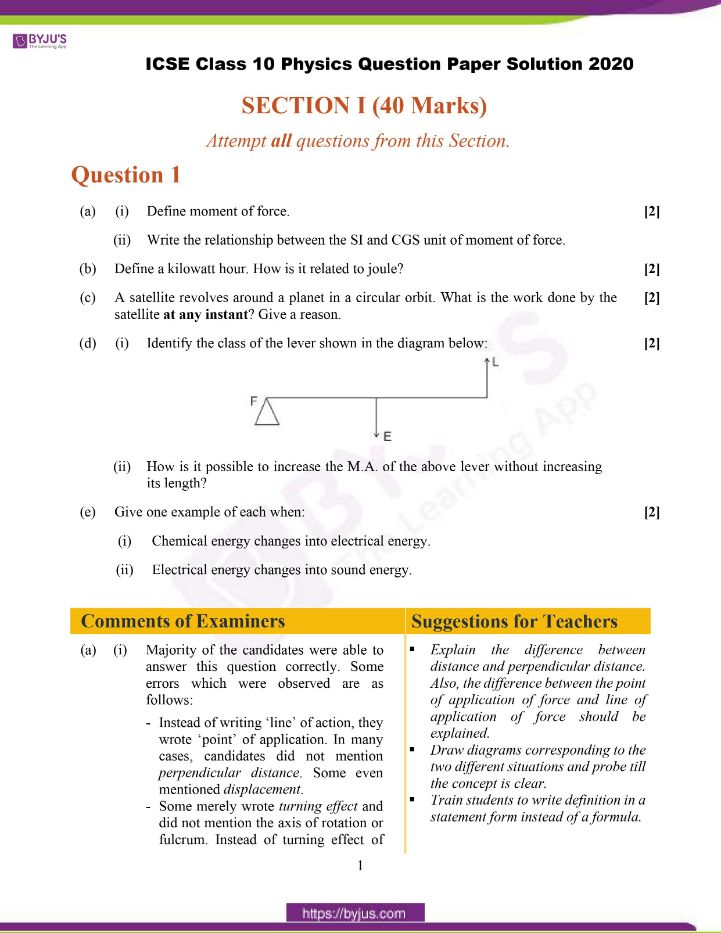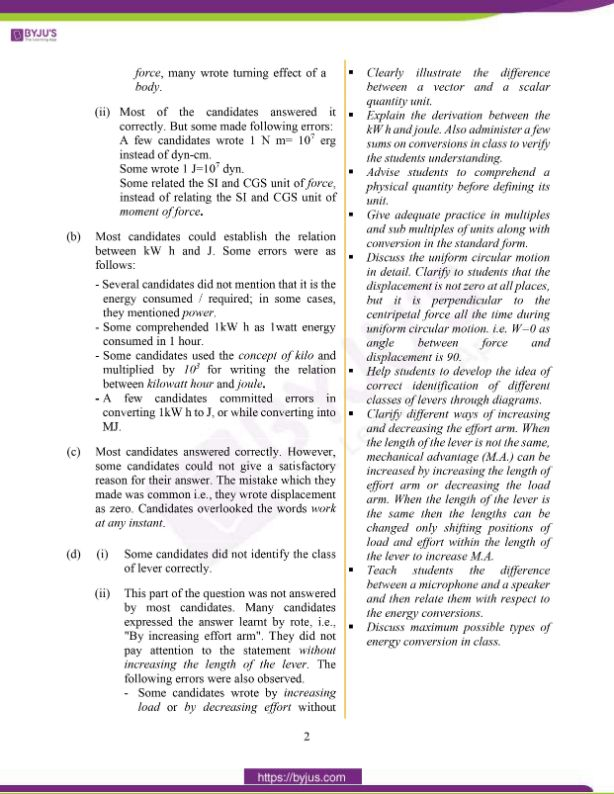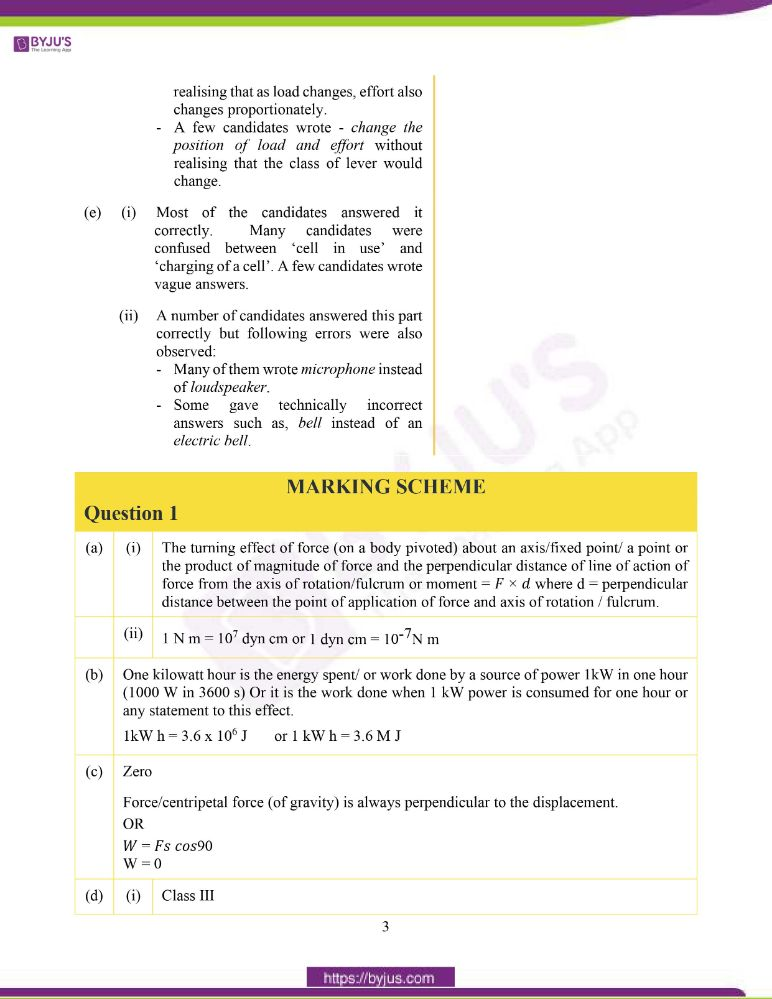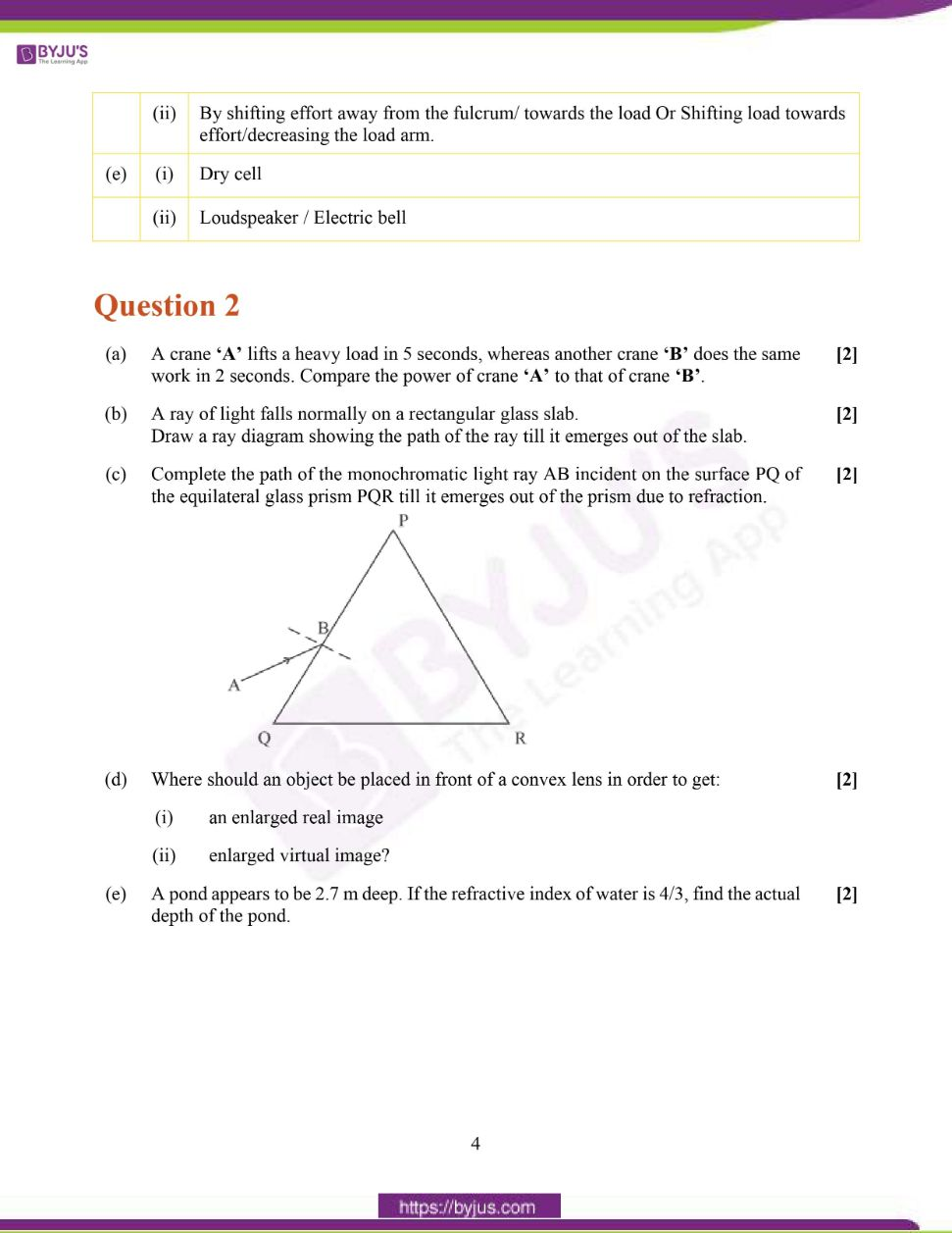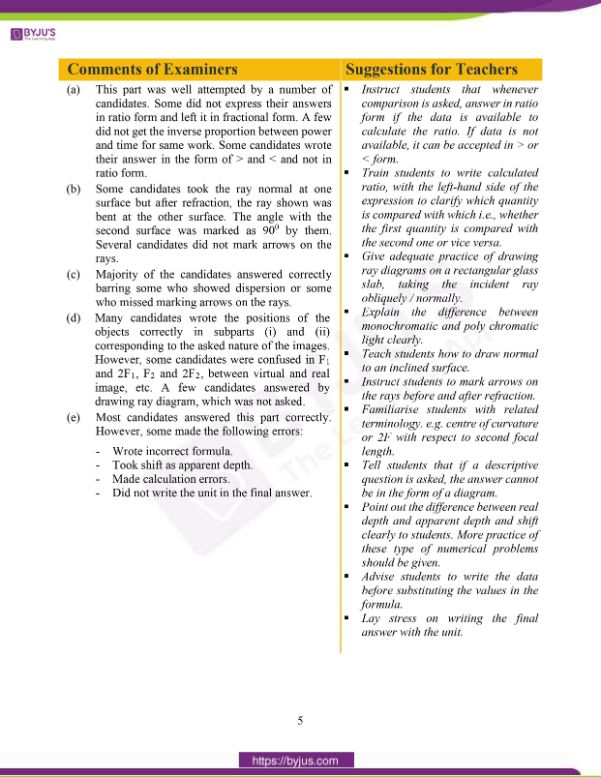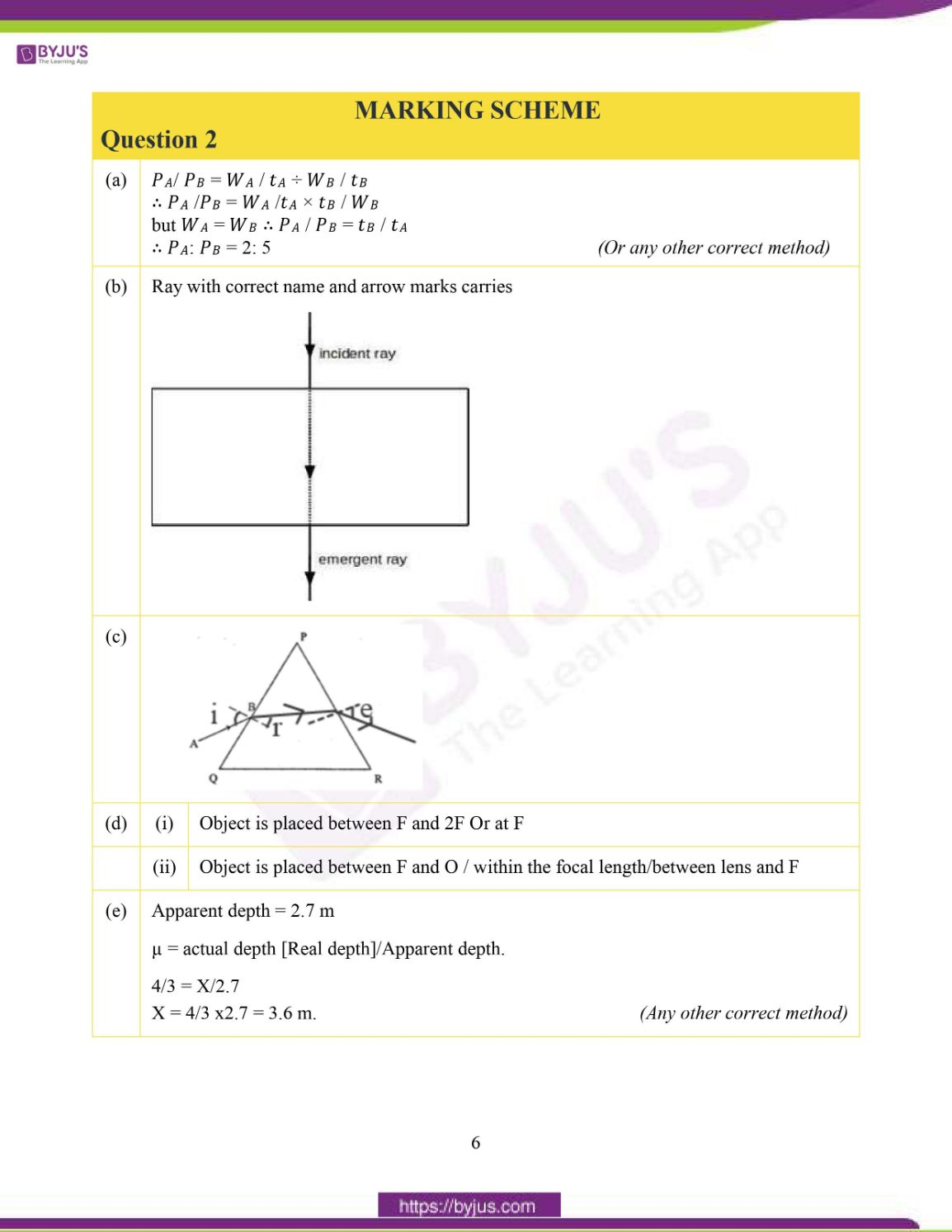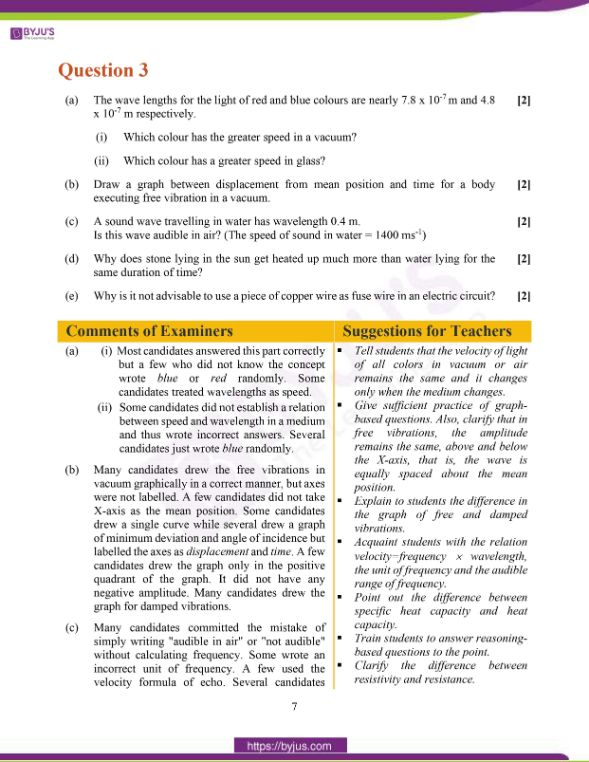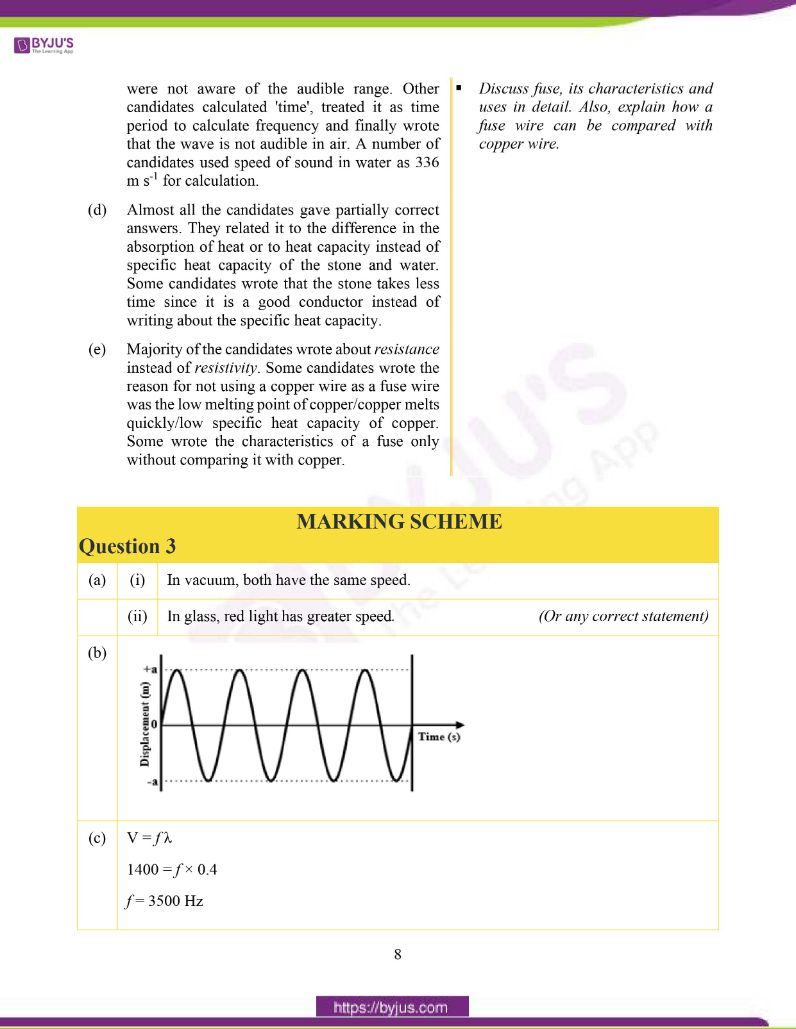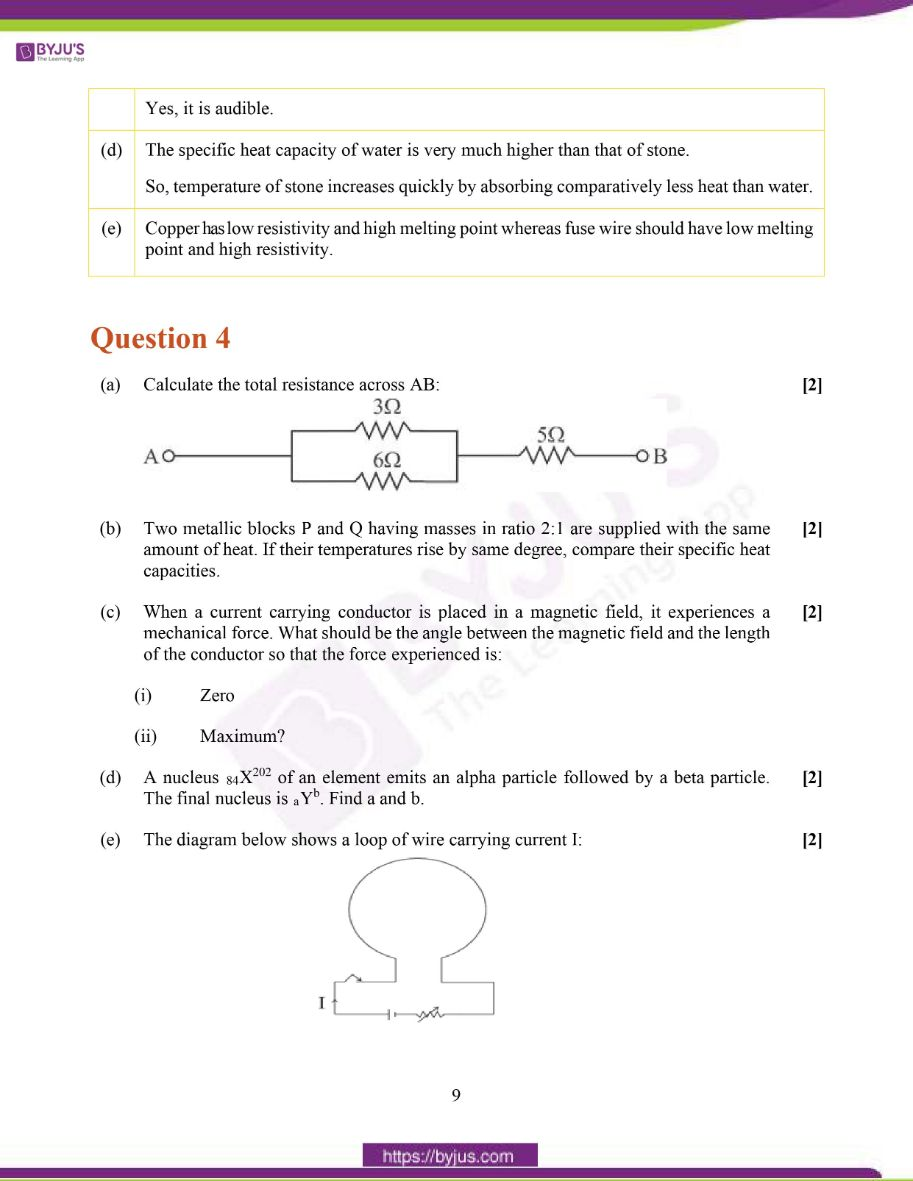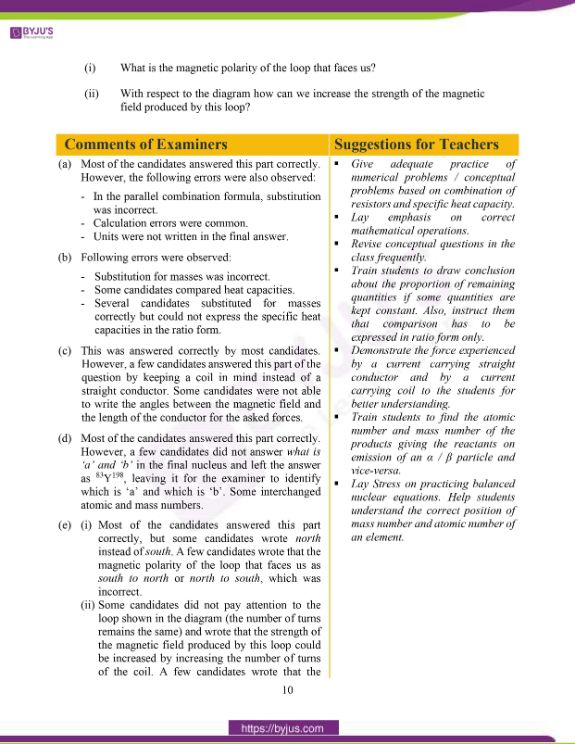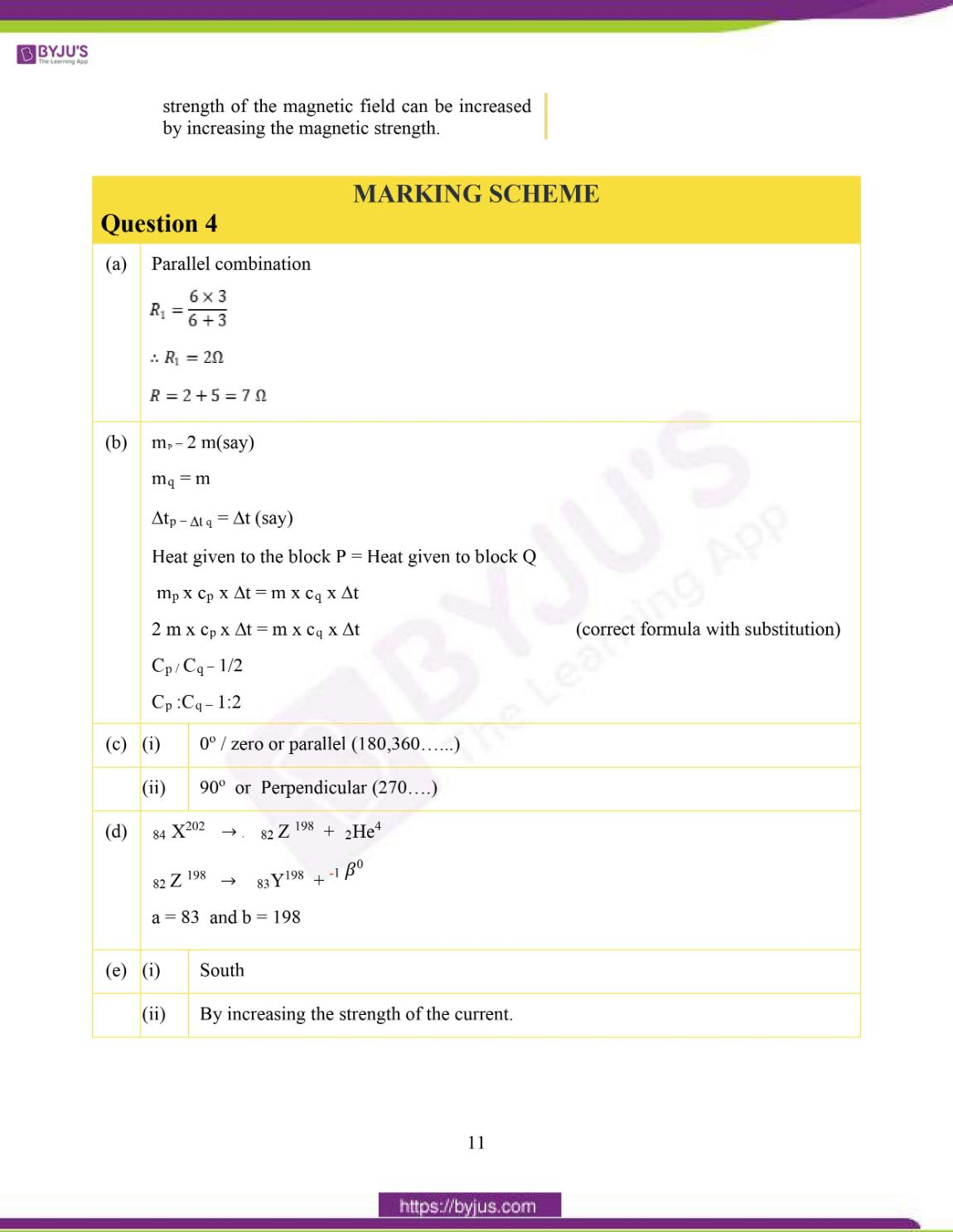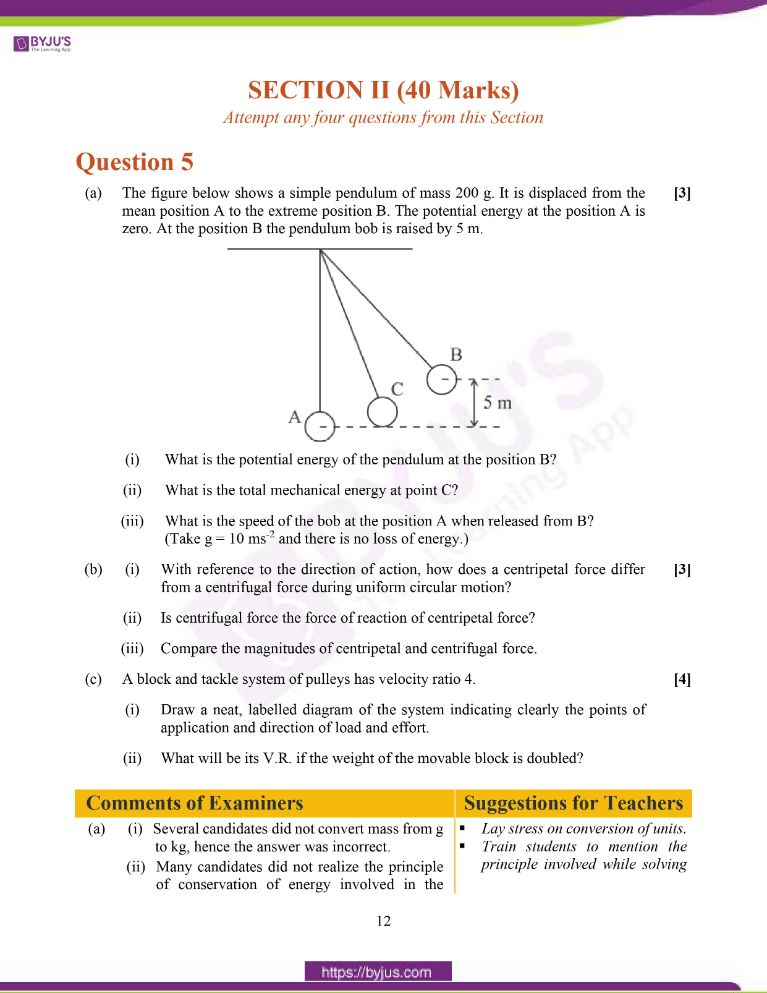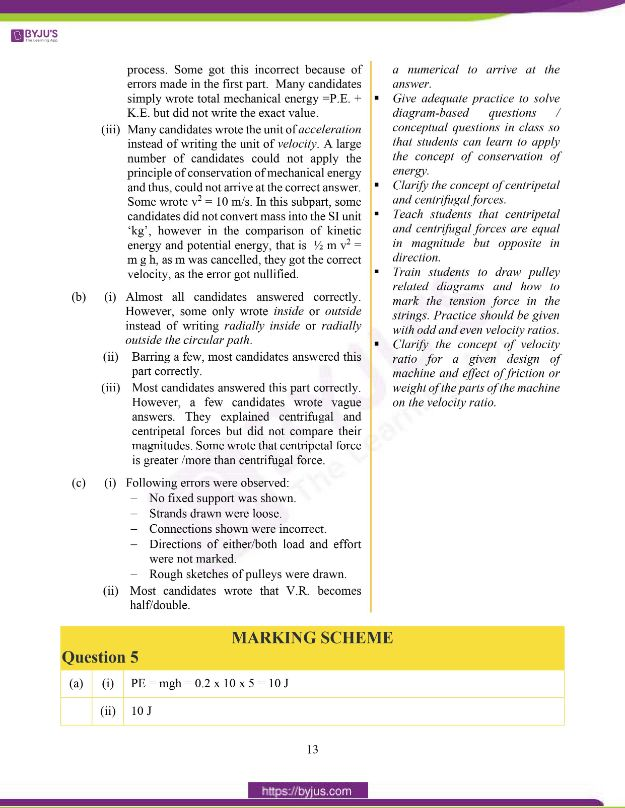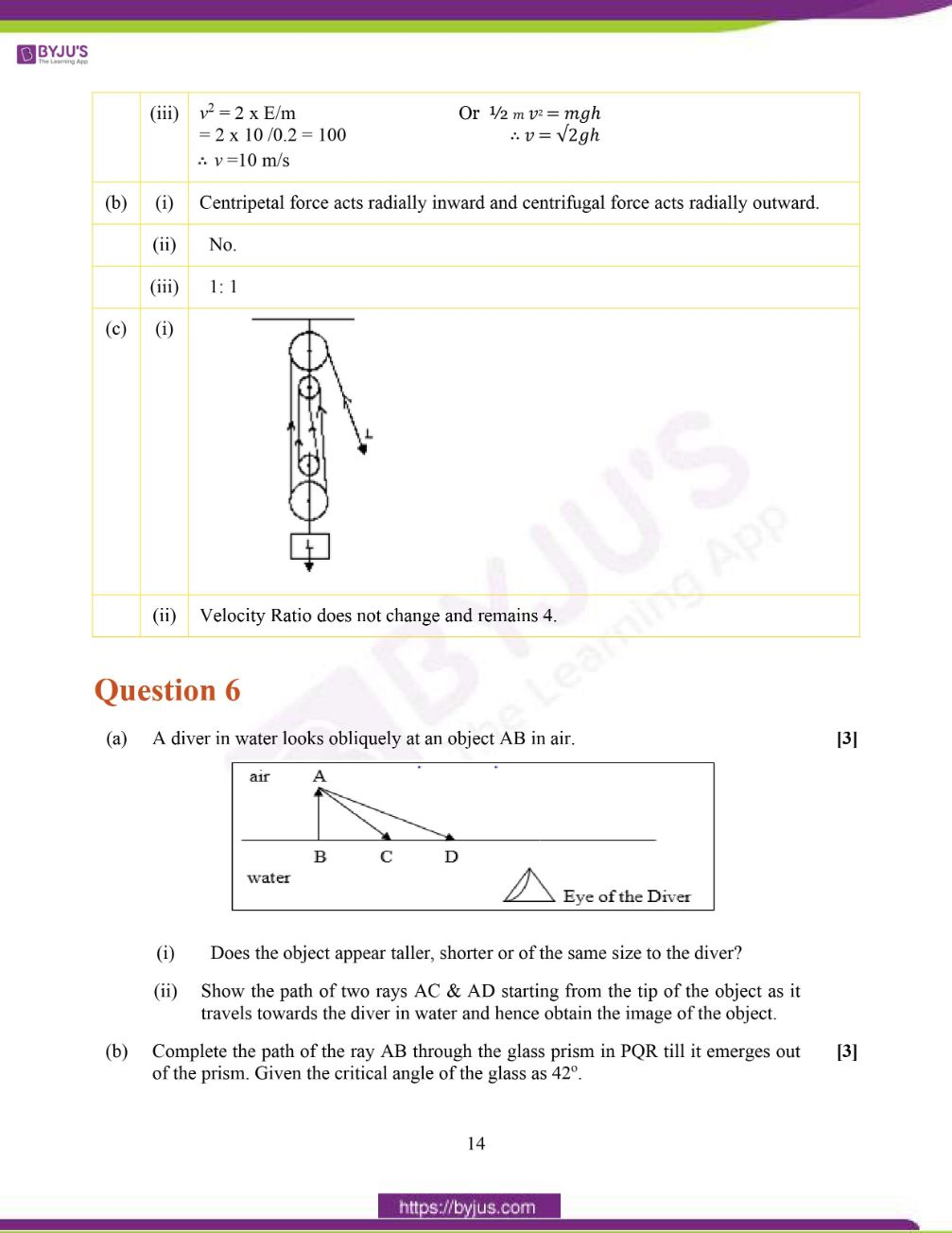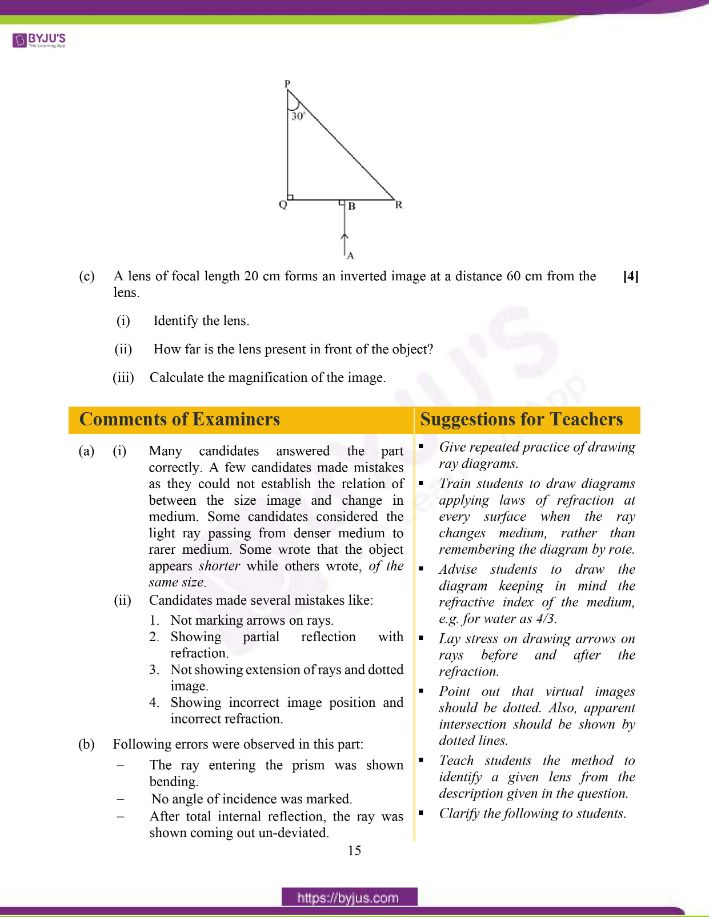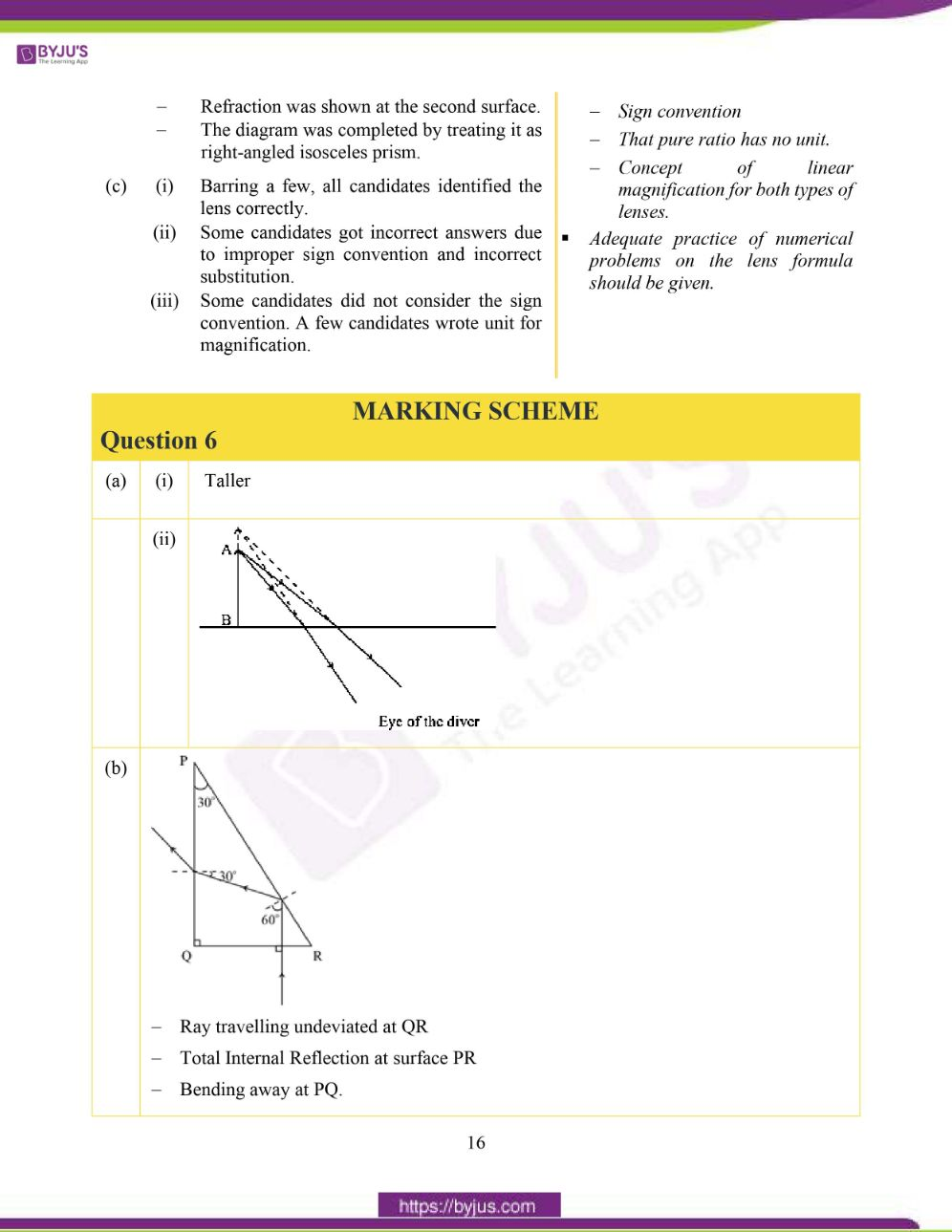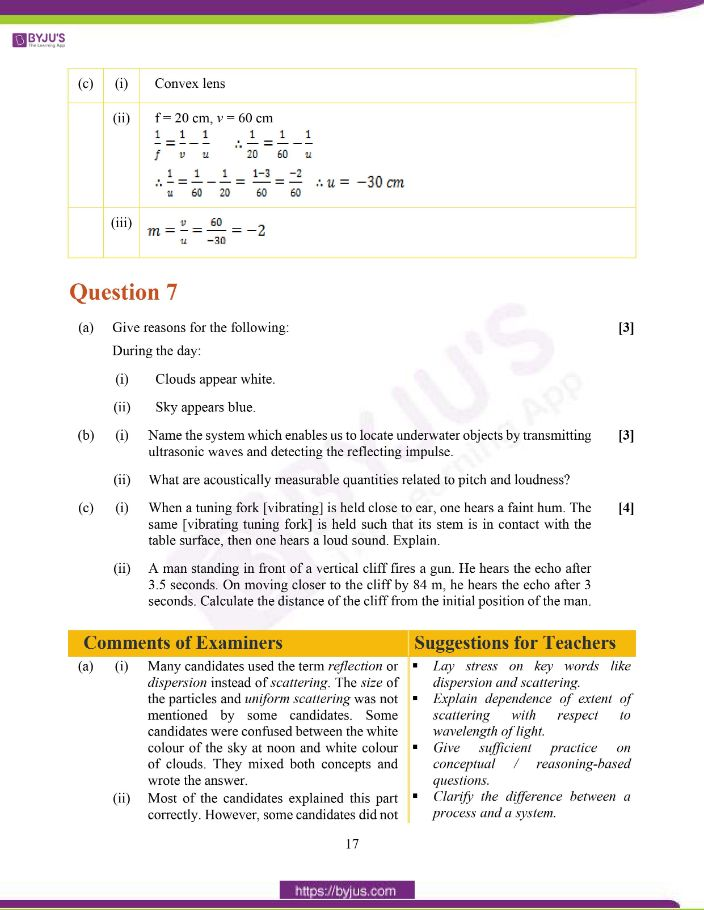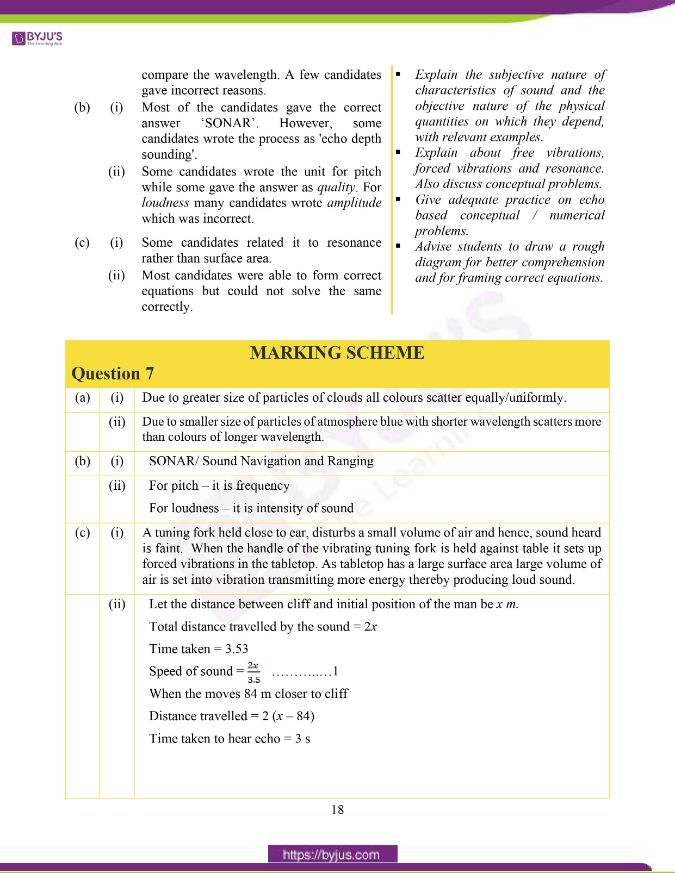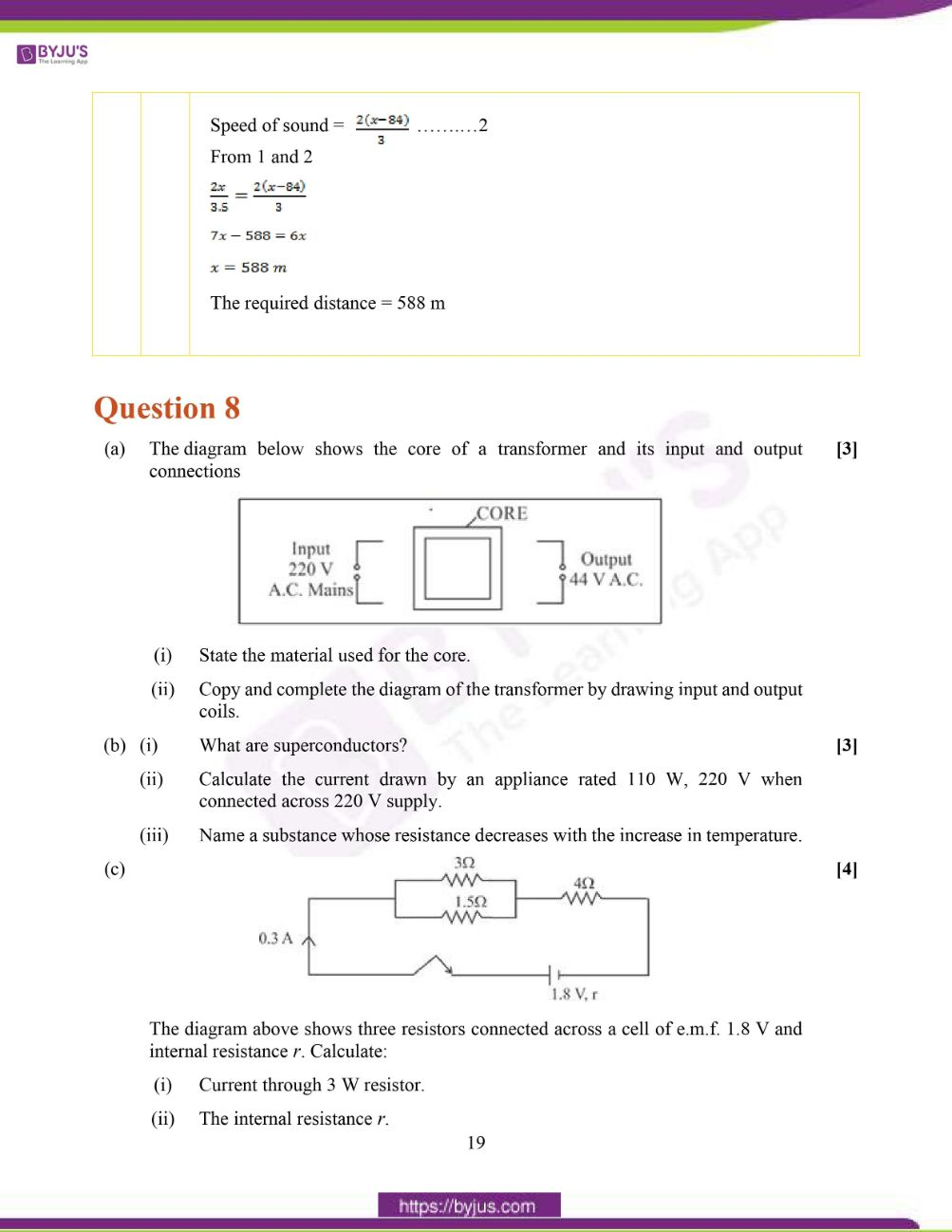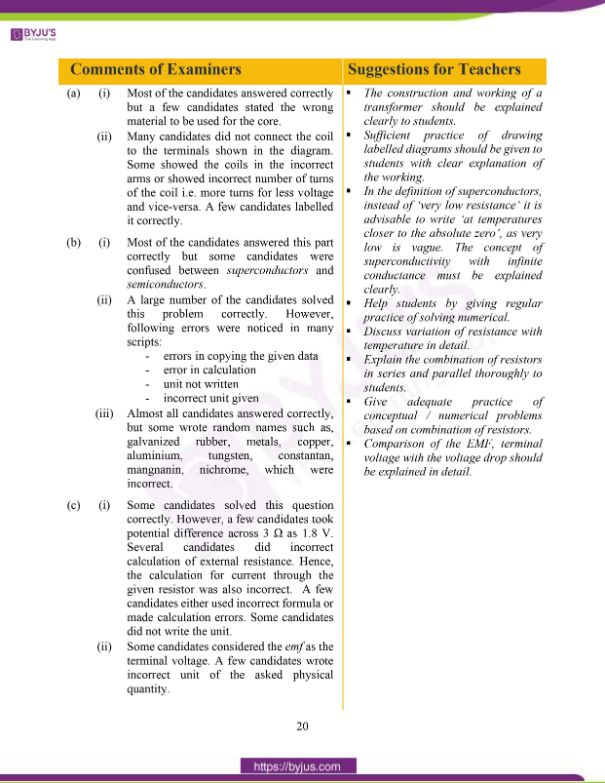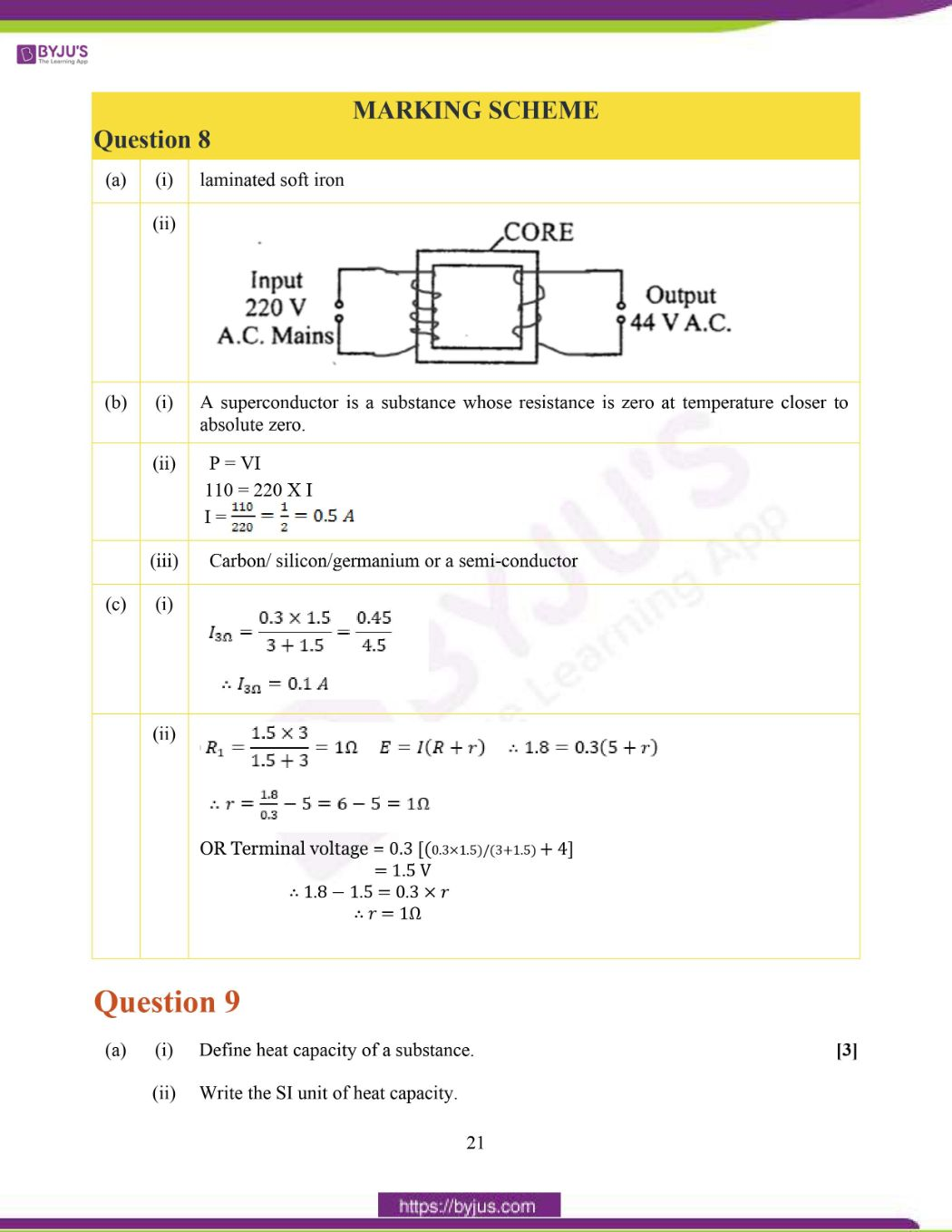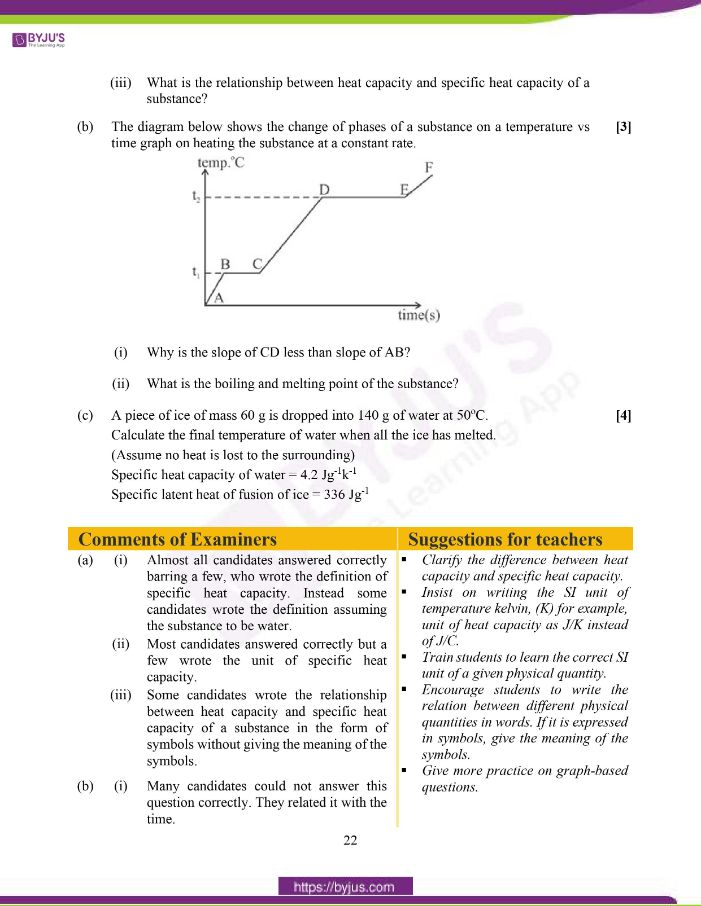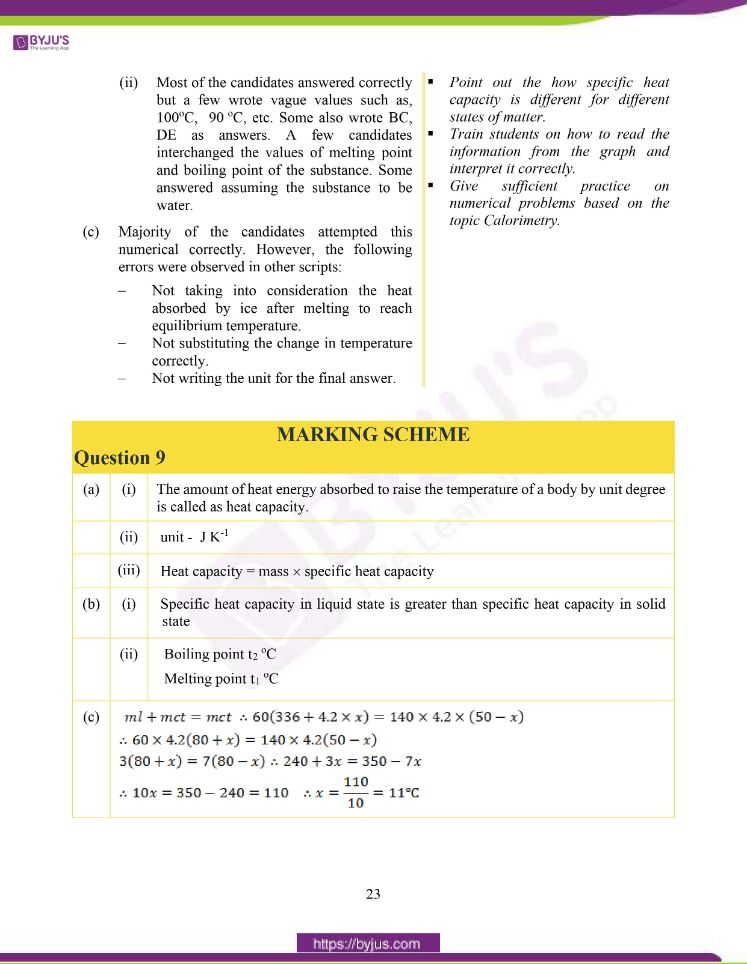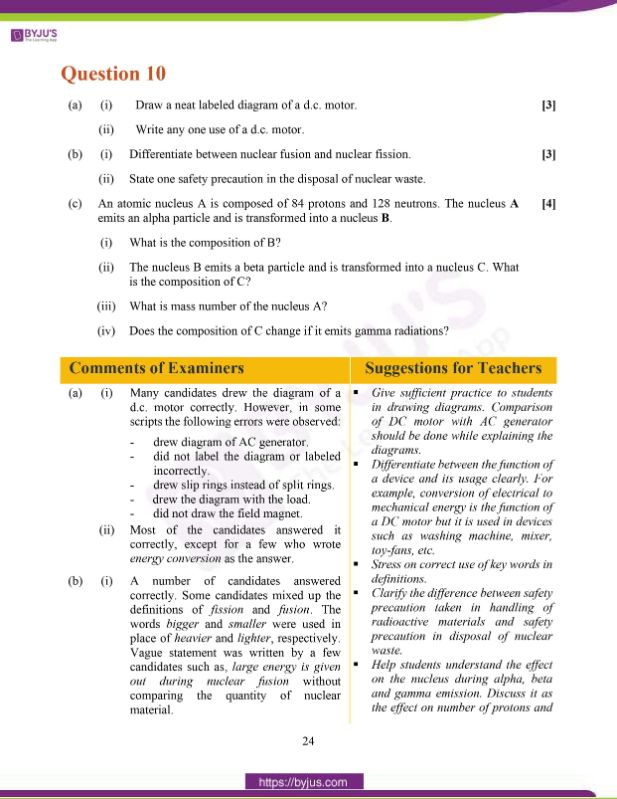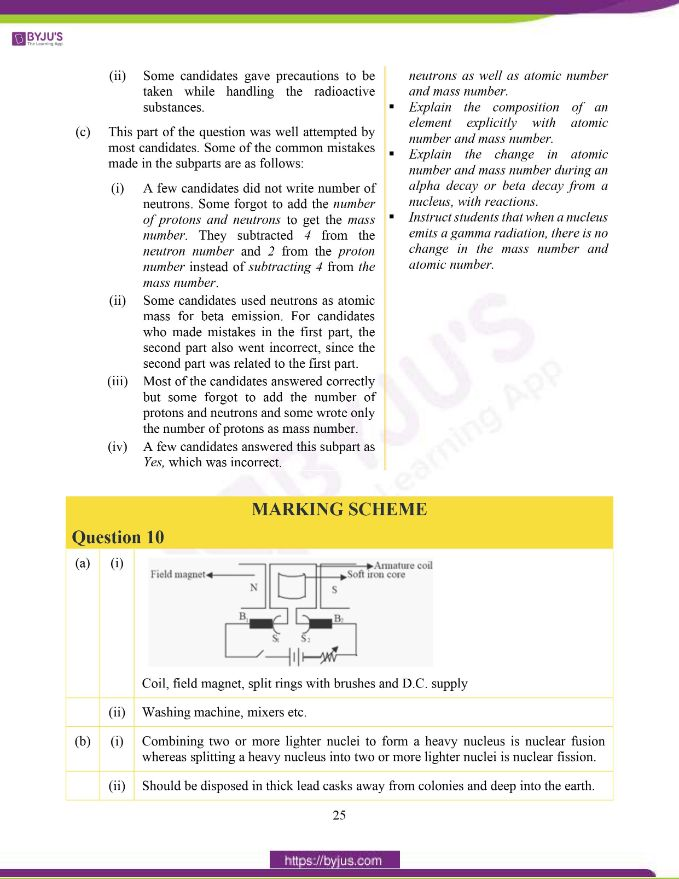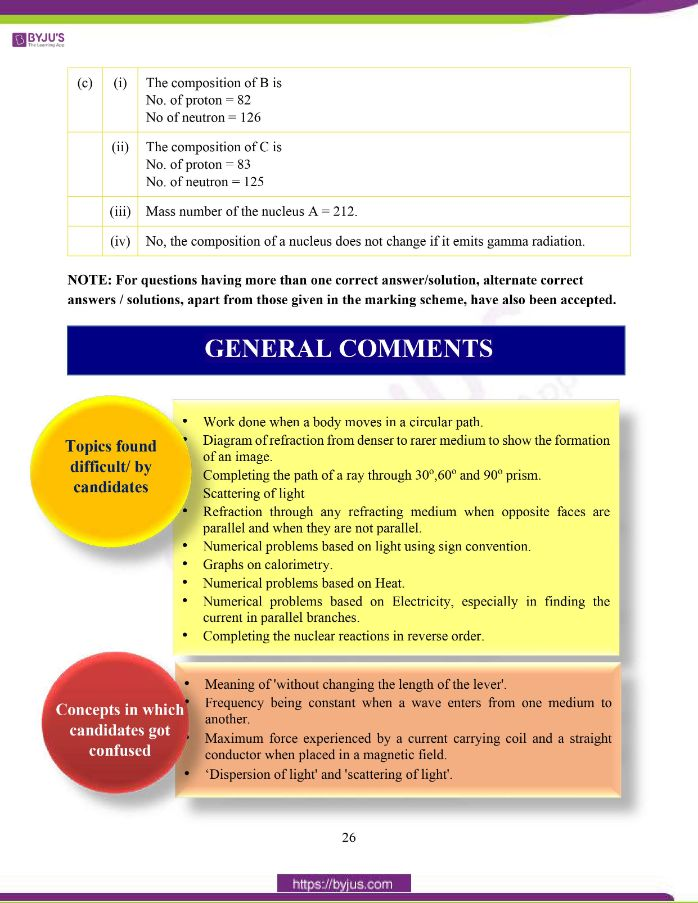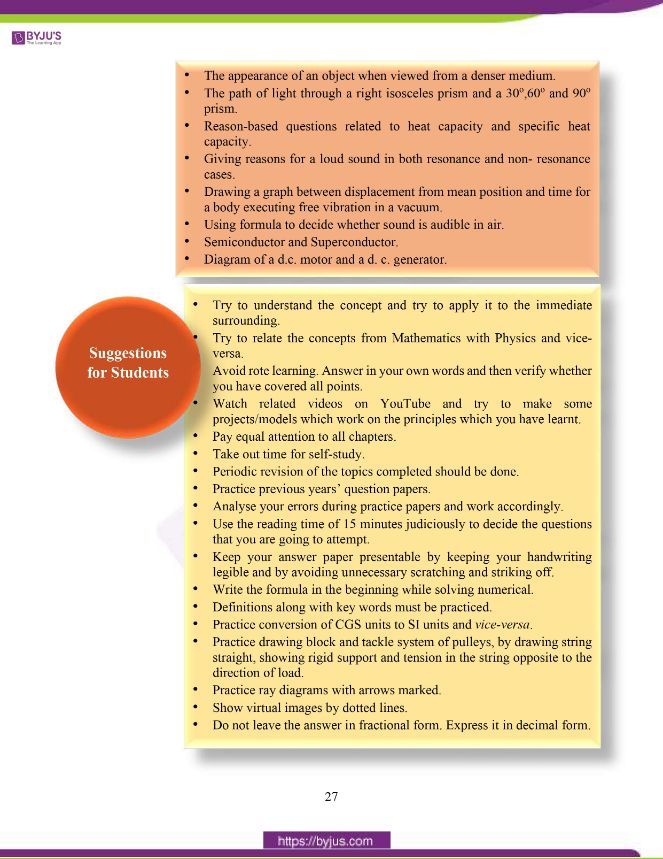### Difficult Topics of ICSE Class 10 Physics 2020 Question Paper

Below we have listed down the topics which students found difficult while attempting the ICSE 2020 Physics Question Paper.

• Work done when a body moves in a circular path.
• Diagram of refraction from denser to rarer medium to show the formation of an image.
• Completing the path of a ray through 30o,60o and 90o prism.
• Scattering of light.
• Refraction through any refracting medium when opposite faces are parallel and when they are not parallel.
• Numerical problems based on light using sign convention.
• Graphs on calorimetry.
• Numerical problems based on Heat.
• Numerical problems based on Electricity, especially in finding the current in parallel branches.
• Completing the nuclear reactions in reverse order.

### Confusing Topics of ICSE Class 10 Physics 2020 Question Paper

Physics concepts in which students got confused while solving the ICSE 2020 Physics question paper.

• Meaning of ‘without changing the length of the lever’.
• Frequency being constant when a wave enters from one medium to another.
• Maximum force experienced by a current carrying coil and a straight conductor when placed in a magnetic field.
• ‘Dispersion of light’ and ‘scattering of light’.
• The appearance of an object when viewed from a denser medium.
• The path of light through a right isosceles prism and a 30o,60o and 90o prism.
• Reason-based questions related to heat capacity and specific heat capacity.
• Giving reasons for a loud sound in both resonance and non- resonance cases.
• Drawing a graph between displacement from mean position and time for a body executing free vibration in a vacuum.
• Using formula to decide whether sound is audible in air.
• Semiconductor and Superconductor.
• Diagram of a d.c. motor and a d. c. generator.

## ICSE Class 10 Physics Question Paper 2020 With Solution

### Question 1:

(a) (i) Define moment of force.

(ii) Write the relationship between the SI and CGS unit of moment of force.

(b) Define a kilowatt hour. How is it related to joule?

(c) A satellite revolves around a planet in a circular orbit. What is the work done by the satellite at any instant? Give a reason.

(d) (i) Identify the class of the lever shown in the diagram below: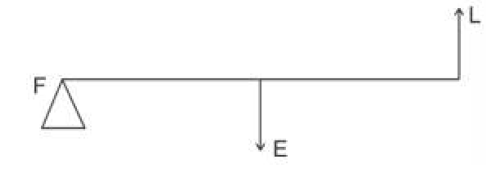(ii) How is it possible to increase the M.A. of the above lever without increasing its length?

(e) Give one example of each when:

(i) Chemical energy changes into electrical energy.

(ii) Electrical energy changes into sound energy.

(a) (i) The turning effect of force (on a body pivoted) about an axis/fixed point/ a point or

the product of magnitude of force and the perpendicular distance of line of action of

force from the axis of rotation/fulcrum or moment = 𝐹 × 𝑑 where d = perpendicular

distance between the point of application of force and axis of rotation / fulcrum.

(ii) 1 N m = 107 dyn cm or 1 dyn cm = 10-7N m

(b) One kilowatt hour is the energy spent/ or work done by a source of power 1kW in one hour (1000 W in 3600 s) Or it is the work done when 1 kW power is consumed for one hour or

any statement to this effect.

1kW h = 3.6 x 106 J or 1 kWh = 3.6 M J

(c) Zero

Force/centripetal force (of gravity) is always perpendicular to the displacement.

OR

W = FS Cos90

W = 0

(d) (i) Class III

(ii) By shifting effort away from the fulcrum/ towards the load Or Shifting load towards effort/decreasing the load arm.

(e) (i) Dry cell

(ii) Loudspeaker / Electric bell

### Question 2:

(a) A crane ‘A’ lifts a heavy load in 5 seconds, whereas another crane ‘B’ does the same work in 2 seconds. Compare the power of crane ‘A’ to that of crane ‘B’.

(b) A ray of light falls normally on a rectangular glass slab. Draw a ray diagram showing the path of the ray till it emerges out of the slab.

(c) Complete the path of the monochromatic light ray AB incident on the surface PQ of the equilateral glass prism PQR till it emerges out of the prism due to refraction.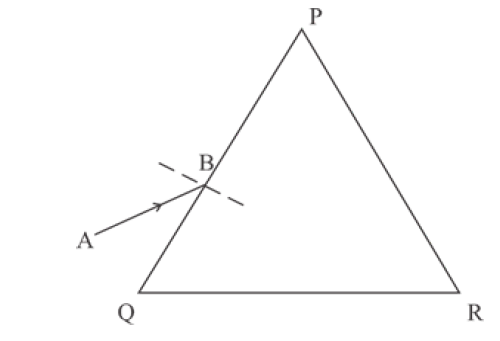(d) Where should an object be placed in front of a convex lens in order to get:

(i) an enlarged real image

(ii) enlarged virtual image?

(e) A pond appears to be 2.7 m deep. If the refractive index of water is 4/3, find the actual depth of the pond.

(a)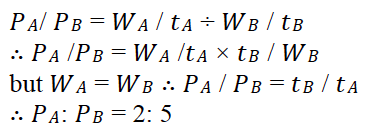(b) Ray with correct name and arrow marks carries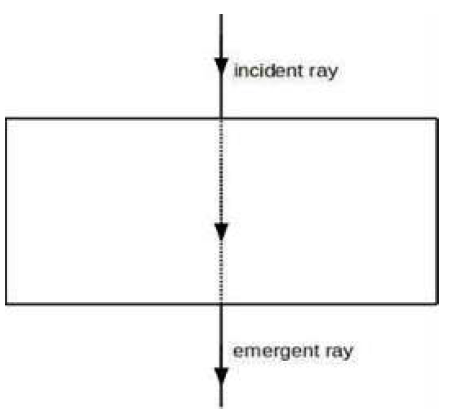(c)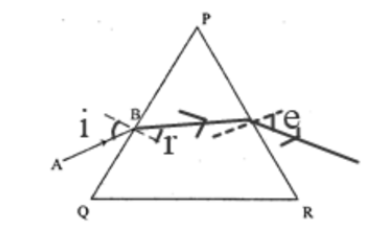(d) (i) Object is placed between F and 2F Or at F

(ii) Object is placed between F and O / within the focal length/between lens and F

(e) Apparent depth = 2.7 m

μ = actual depth [Real depth]/Apparent depth.

4/3 = X/2.7

X = 4/3 x2.7 = 3.6 m.

### Question 3:

(a) The wave lengths for the light of red and blue colours are nearly 7.8×10-7 m and 4.8×10-7 m, respectively.

(i) Which colour has the greater speed in a vacuum?

(ii) Which colour has a greater speed in glass?

(b) Draw a graph between displacement from mean position and time for a body executing free vibration in a vacuum.

(c) A sound wave travelling in water has a wavelength 0.4 m. Is this wave audible in air? (The speed of sound in water = 1400 ms-1)

(d) Why does stone lying in the sun get heated up much more than water lying for the same duration of time?

(e) Why is it not advisable to use a piece of copper wire as fuse wire in an electric circuit?

(a) (i) In vacuum, both have the same speed.

(ii) In glass, red light has greater speed.

(b)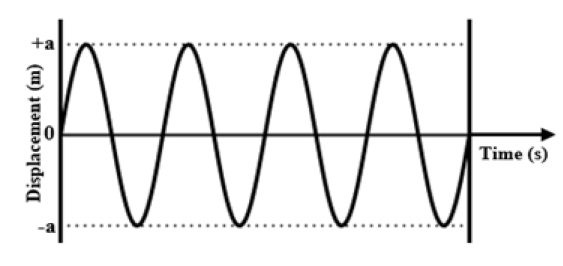(c) V = f λ

1400 = f ×0.4

f = 3500 Hz

Yes, it is audible.

(d) The specific heat capacity of water is very much higher than that of stone. So, the temperature of stone increases quickly by absorbing comparatively less heat than water.

(e) Copper has low resistivity and high melting point whereas fuse wire should have low melting

point and high resistivity.

### Question 4:

(a) Calculate the total resistance across AB: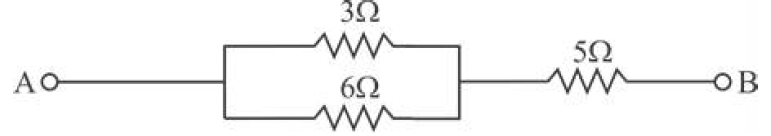(b) Two metallic blocks P and Q having masses in ratio 2:1 are supplied with the same amount of heat. If their temperatures rise by the same degree, compare their specific heat capacities.

(c) When a current carrying conductor is placed in a magnetic field, it experiences a mechanical force. What should be the angle between the magnetic field and the length of the conductor so that the force experienced is:

(i) Zero

(ii) Maximum?

(d) A nucleus 84X202 of an element emits an alpha particle followed by a beta particle. The final nucleus is aYb. Find a and b.

(e) The diagram below shows a loop of wire carrying current I: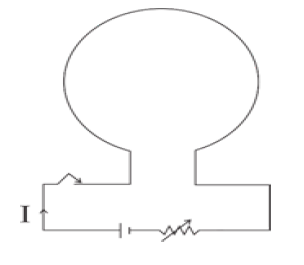(i) What is the magnetic polarity of the loop that faces us?

(ii) With respect to the diagram how can we increase the strength of the magnetic field produced by this loop?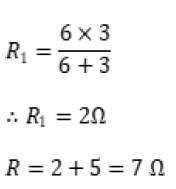(b) mP = 2 m (say)

mq = m

Δtp = Δtq = Δt (say)

Heat given to the block P = Heat given to block Q

mp x cp x Δt = m x cq x Δt

2 m x cp x Δt = m x cq x Δt (correct formula with substitution)

Cp / Cq = 1/2

Cp :Cq = 1:2

(c) (i) 0o / zero or parallel (180, 360……)

(ii) 90o or Perpendicular (270….)

(d) 84 X20282 Z 198 + 2He4

82 Z 19883Y198 + –1𝛽0

a = 83 and b = 198

(e) (i) South

(ii) By increasing the strength of the current.

### Question 5:

(a) The figure below shows a simple pendulum of mass 200 g. It is displaced from the mean position A to the extreme position B. The potential energy at position A is zero. At the position B the pendulum bob is raised by 5 m.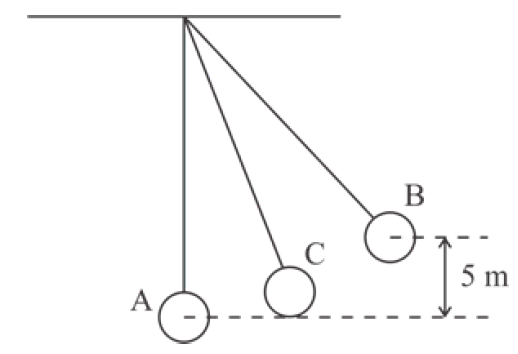(i) What is the potential energy of the pendulum at position B?

(ii) What is the total mechanical energy at point C?

(iii) What is the speed of the bob at the position A when released from B?

(Take g = 10 ms-2 and there is no loss of energy.)

(b) (i) With reference to the direction of action, how does a centripetal force differ

from a centrifugal force during uniform circular motion?

(ii) Is centrifugal force the force of reaction of centripetal force?

(iii) Compare the magnitudes of centripetal and centrifugal force.

(c) A block and tackle system of pulleys has velocity ratio 4.

(i) Draw a neat, labelled diagram of the system indicating clearly the points of application and direction of load and effort.

(ii) What will be its V.R. if the weight of the movable block is doubled?

(a) (i) PE = mgh = 0.2 x 10 x 5 = 10 J

(ii) 10 J

(iii)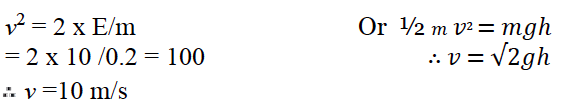(b) (i) Centripetal force acts radially inward and centrifugal force acts radially outward.

(ii) No.

(iii) 1: 1

(c)(i)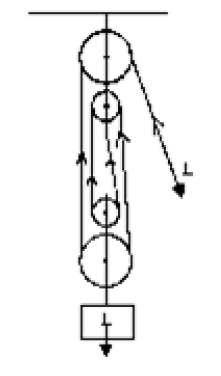(ii) Velocity Ratio does not change and remains 4.

### Question 6:

(a) A diver in water looks obliquely at an object AB in the air.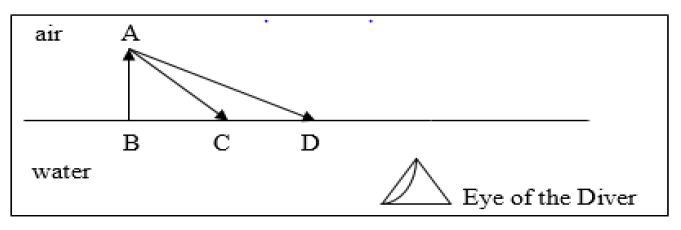(i) Does the object appear taller, shorter or of the same size to the diver?

(ii) Show the path of two rays AC & AD starting from the tip of the object as it travels towards the diver in water and hence obtains the image of the object.

(b) Complete the path of the ray AB through the glass prism in PQR till it emerges out of the prism. Given the critical angle of the glass as 42o.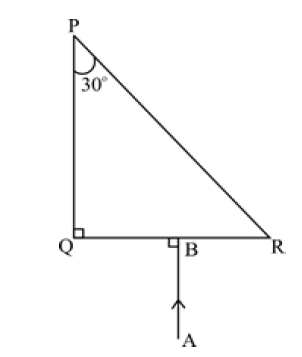(c) A lens of focal length 20 cm forms an inverted image at a distance 60 cm from the lens.

(i) Identify the lens.

(ii) How far is the lens present in front of the object?

(iii) Calculate the magnification of the image.

(ii)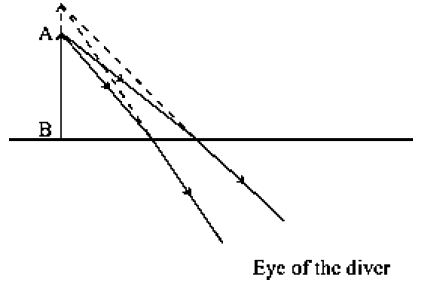(b)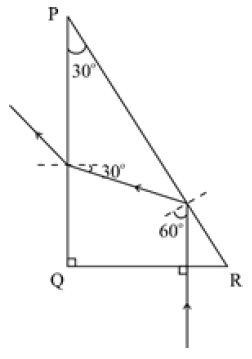− Ray travelling undeviated at QR

− Total Internal Reflection at surface PR

− Bending away at PQ.

(c) (i) Convex lens

(ii) f = 20 cm, v = 60 cm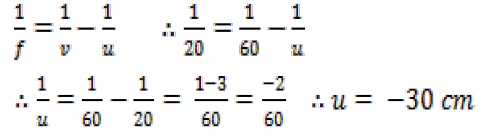(iii) m = v/u = 60/-30 = -2

### Question 7:

(a) Give reasons for the following:

During the day:

(i) Clouds appear white.

(ii) Sky appears blue.

(b) (i) Name the system which enables us to locate underwater objects by transmitting ultrasonic waves and detecting the reflecting impulse.

(ii) What are acoustically measurable quantities related to pitch and loudness?

(c) (i) When a tuning fork [vibrating] is held close to the ear, one hears a faint hum. The same [vibrating tuning fork] is held such that its stem is in contact with the table surface, then one hears a loud sound. Explain.

(ii) A man standing in front of a vertical cliff fires a gun. He hears the echo after 3.5 seconds. On moving closer to the cliff by 84 m, he hears the echo after 3 seconds. Calculate the distance of the cliff from the initial position of the man.

(a) (i) Due to greater size of particles of clouds all colours scatter equally/uniformly.

(ii) Due to smaller size of particles of atmosphere blue with shorter wavelength scatters more than colours of longer wavelength.

(b) (i) SONAR/ Sound Navigation and Ranging

(ii) For pitch – it is frequency

For loudness – it is intensity of sound

(c) (i) A tuning fork held close to the ear disturbs a small volume of air and hence, sound heard is faint. When the handle of the vibrating tuning fork is held against the table it sets up forced vibrations in the tabletop. As the tabletop has a large surface area a large volume of air is set into vibration transmitting more energy thereby producing loud sound.

(ii) Let the distance between the cliff and the initial position of the man be x m.

Total distance travelled by the sound = 2x

Time taken = 3.53

Speed of sound = 2x/3.5………..…1

When the moves 84 m closer to cliff

Distance travelled = 2 (x – 84)

Time taken to hear echo = 3 s

Speed of sound = 2(x-84)/3 …….…2

From 1 and 2

2x/3.5 = 2(x-84)/3

7x – 588 = 6x

x = 588 m

The required distance = 588 m

### Question 8:

(a) The diagram below shows the core of a transformer and its input and output connections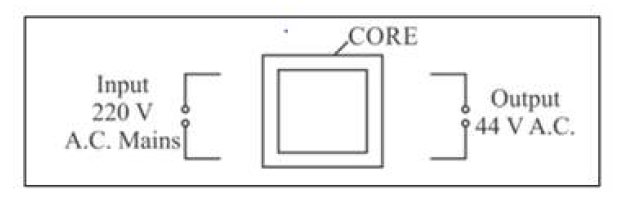(i) State the material used for the core.

(ii) Copy and complete the diagram of the transformer by drawing input and output coils.

(b) (i) What are superconductors?

(ii) Calculate the current drawn by an appliance rated 110 W, 220 V when connected across 220 V supply.

(iii) Name a substance whose resistance decreases with the increase in temperature.

(c)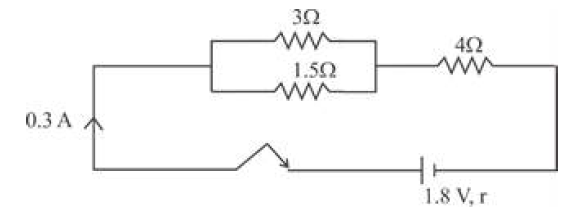The diagram above shows three resistors connected across a cell of e.m.f. 1.8 V and internal resistance r. Calculate:

(i) Current through 3 W resistor.

(ii) The internal resistance r.

Answer: (a) (i) laminated soft iron

(ii)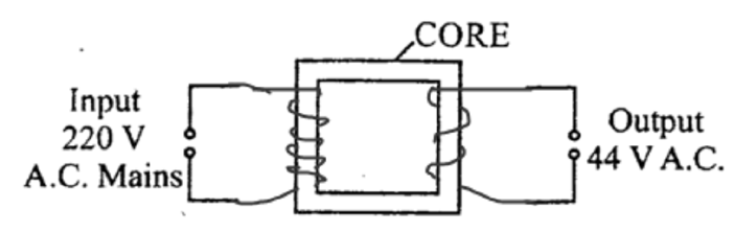(b) (i) A superconductor is a substance whose resistance is zero at temperature closer to

absolute zero.

(ii) P = VI

110 = 220 X I

I = 110/220 = 1/2 = 0.5 A

(iii) Carbon/ silicon/germanium or a semiconductor

(c) (i)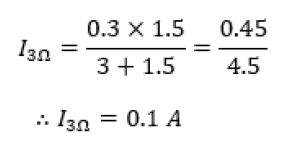(ii)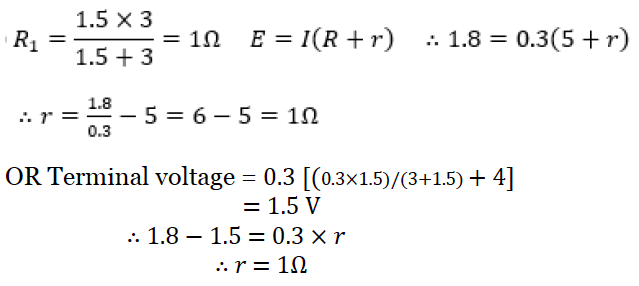### Question 9:

(a) (i) Define heat capacity of a substance.

(ii) Write the SI unit of heat capacity.

(iii) What is the relationship between heat capacity and specific heat capacity of a substance?

(b) The diagram below shows the change of phases of a substance on a temperature vs time graph on heating the substance at a constant rate.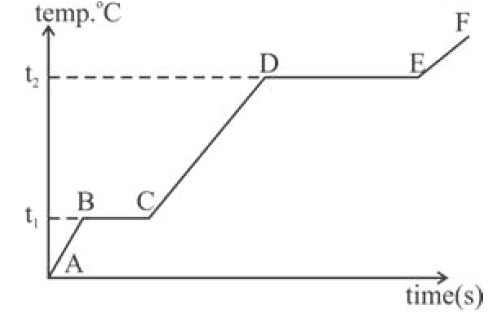(i) Why is the slope of CD less than the slope of AB?

(ii) What is the boiling and melting point of the substance?

(c) A piece of ice of mass 60 g is dropped into 140 g of water at 50oC. Calculate the final temperature of water when all the ice has melted.

(Assume no heat is lost to the surrounding)

Specific heat capacity of water = 4.2 Jg-1k-1

Specific latent heat of fusion of ice = 336 Jg-1

(a) (i) The amount of heat energy absorbed to raise the temperature of a body by unit degree is called heat capacity.

(ii) unit: J K-1

(iii) Heat capacity = mass × specific heat capacity

(b) (i) Specific heat capacity in liquid state is greater than specific heat capacity in solid state

(ii) Boiling point t2 oC

Melting point t1 oC

(c)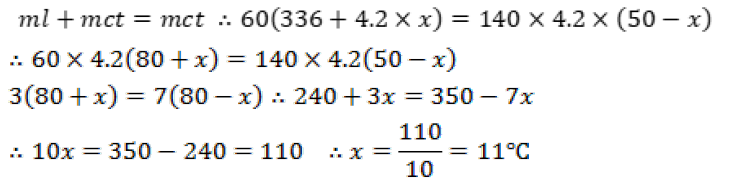### Question 10:

(a) (i) Draw a neat labeled diagram of a d.c. motor.

(ii) Write any one use of a d.c. motor.

(b) (i) Differentiate between nuclear fusion and nuclear fission.

(ii) State one safety precaution in the disposal of nuclear waste.

(c) An atomic nucleus A is composed of 84 protons and 128 neutrons. The nucleus A emits an alpha particle and is transformed into a nucleus B.

(i) What is the composition of B?

(ii) The nucleus B emits a beta particle and is transformed into a nucleus C. What is the composition of C?

(iii) What is the mass number of the nucleus A?

(iv) Does the composition of C change if it emits gamma radiations?

(a) (i)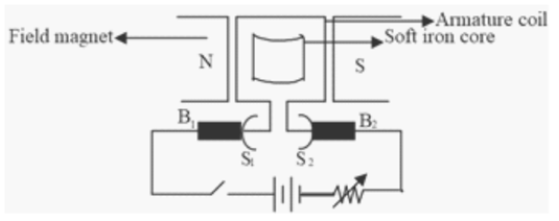Coil, field magnet, split rings with brushes and D.C. supply

(ii) Washing machine, mixers, etc.

(b) (i) Combining two or more lighter nuclei to form a heavy nucleus is nuclear fusion

whereas splitting a heavy nucleus into two or more lighter nuclei is nuclear fission.

(ii) Should be disposed of in thick lead casks away from colonies and deep into the earth.

(c) (i) The composition of B is

No. of proton = 82

No of neutron = 126

(ii) The composition of C is

No. of proton = 83

No. of neutron = 125

(iii) Mass number of the nucleus A = 212.

(iv) No, the composition of a nucleus does not change if it emits gamma radiation.

NOTE: For questions having more than one correct answer/solution, alternative correct answers / solutions, apart from those given in the marking scheme, have also been accepted.

We hope students must have found this information on “ICSE Class 10 Physics Question Papers Solutions 2020” helpful for their studies. To get the year wise ICSE Class 10 Previous Years Question papers along with solutions for other subjects, click here. Keep learning and download the BYJU’S App to access interactive study videos.